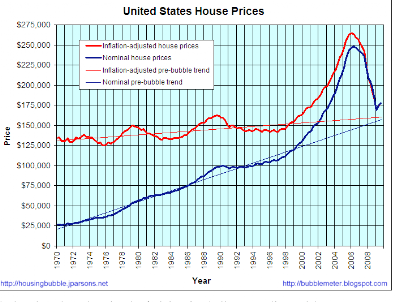Thursday, March 18, 2010

Rental vacancies and house prices

The first figure below is from a simulation of a toy economy I ran based on Steve Keen's model.I detected an obvious relationship between rental vacancies and house prices. The rental vacancy peaks ahead of the house price peak and occurs at about midpoint between the house price bottoms and tops. After the rental vacancy peaks, the house prices increase until the rental vacancy reaches a low level. The rental vacancy approaching a bottom is an indicator of a house price top.

I wondered if this relationship held up in the real world. The second and third figures show US house prices and rental vacancies.The house price shows a bottom in 1976 and a top in 1979. The rental vacancy peaks at 6+% in 1975 and declines to 5% in 1978. The rental top and bottom anticipated the house price movement.

The house price then declined from 1979 to 1983. The rental vacancy then blipped up a bit from 1979 to 1981 and then declined to a relative bottom in 1981-82. Again, the rental vacancy anticipated the house prices.

Beginning in 1982, the rental vacancy rose steadily from 5% to a peak in 1988 of 8%. The rental vacancy then declined from 1988 to 1990 to another relative bottom. The house prices during this time bottomed in 1983 and rose to a top in 1990. The peak rental vacancy anticipated the price top by 2 years.

The rental vacancy bottomed at slightly under 7% in 1994 and then rose gradually until 2001. The house price during this time bottomed in 1996-97 and then climbed steeply to a top in 2006. The top of the house price rise was anticipated by the rental vacancy peak of 10+% of 2004. The relative bottom of rental vacancy was simultaneous with the price top in 2006.

I conclude that the rental vacancy-house price relationship predicted by the simulation is substantiated by the empirical data.

The program code is attached for interested SciLabbers.

z0=[500;500;0;0;1;1;1;1000;0;0;0;500;20;20;1;1;80;10;10;1;100];
t=0:1:200;
clf()

function dx = keeninterm(t,x)

rL=.05
rD=.03
s=.3

tau_HQ=1

v=5
HV=26/4

sH=0.3

tauS=1
tauW=1/(26-HV)

tau_Q=1;tau_L=1
tau_H=1

tau_pi=1,tau_piH=1

tausH=8;tauWH=1/HV;

//if t> 160 then
//fac=.95;
//else fac=1.0;
//end
//if t>190 then
//fac=1.0
//end
fac=1

tauL=7*fac
tauR=1/fac
tauM=16/fac
tauMH=16/fac

tauB=1.25;tauBH=5
tauP=1

alpha=.015;alphaH=.015;
bta=.02

gma=.01;gmaH=.01

stimH=0
//if t>75 then
//stimH=.4
//end

A=rL*x(1); B=A; C=rD*x(2);D=(1-s)/tauS*x(2);E=rD*x(3);F=x(3)/tauW;G=x(4)/tauB;
H=x(1)/tauL;
I=x(9)*x(2)/((x(2)+x(14))*tauR);
J=x(2)/tauM;

HA=rL*x(13);HB=HA;HC=rD*x(14);HD=(1-sH)/tausH*x(14);HF=x(3)/tauWH;HH=x(13)/tauL;HI=x(9)*x(14)/((x(2)+x(14))*tauR);HJ=x(14)/tauMH
HG=x(4)/tauBH;LT=x(12)+x(19);c=x(18)/LT;vacancy=x(21)-(LT-x(18))

//loss=x(20)*vacancy*x(21)/(x(21)-vacancy)
loss=0
HK=x(17)-loss

CF=1-2*c+stimH

SS=c*(1-c)*LT^2
SSmax=.25*LT^2

if (c^2)^.5 <1 ssrat="SS/SSmax" ssrat="1" ge="genexp(x,xv,yv,s,m)" ge="(yv-m)*exp(s/(yv-m)*(x-xv))" lamda="(x(12)+x(19))./x(8)" ph="genexp(lamda,.97,0,1.5,-.06)

unemp=1-lamda

dx(1)=A-B-H+I+J
dx(2)=-B+C-D+F+G-H+I+J
dx(3)=D+E-F+HD-HF
dx(4)=B-C-E-G+HB-HC-HG
dx(5)=-1/tauP*(x(5)-x(6)./((1-s)*x(7)))
dx(6)=Ph*x(6)
dx(7)=alpha*x(7)
dx(8)=bta*x(8)
dx(9)=H-I-HI+HH

dx(10)=x(2)/tau_pi-gma*x(10)
dx(10)=x(2)/tauS-gma*x(10)

dx(11)=-.25/tau_Q*(x(11)-x(10)/(v*x(5)))
dx(12)=-1/tau_L*(x(12)-x(11)/x(7))

Profit=x(5)*x(11)-x(6)*x(12)-A+C
ProfitH=x(15)*x(18)-x(6)*x(19)-HA+HC

k=SSrat

k_H=1

dx(13)=HA-HB-HH+HI+HJ

cbar=.10*(.3*x(6)+.7*x(5));
Cbar=.15*(.7*x(6)+.3*x(5));

//ProfitH=HProfit+RProfit

dx(14)=-HB+HC-HD+HF+HG-HH+HI+HJ//+Profit

//HP=HK*(1+.5*exp(-((t-107)/10)^2)-.2*exp(-((t-120)/10)^2))
HP=HK*(1+.5*exp(-((t-107)/10)^2))//-.2*exp(-((t-120)/10)^2))
//HP=HK
//if t>108 then
//HP=HK*(1+.25*exp(-((t-107)/10)^2)-.5*exp(-((t-112)/10)^2))
//end

dx(15)=-k/tauP*(x(15)-(HP-x(20)*x(21))/(10*x(18)))

dx(16)=x(16)*alphaH

dx(17)=x(14)/tausH-gma*x(17)

dx(18)=-CF/tau_HQ*(x(18)-(HK*(1-sH)-cbar*x(21))/Cbar)

dx(19)=-.25/tau_L*(x(19)-(.7*x(18)+.3*x(21))/x(16))

dx(20)=-1/tauP*(x(20)-(HK-x(15)*x(18))/(10*x(21)))

//dx(21)=-.1/tau_HQ*(x(21)+vacancy-(HK*(1-sH)-Cbar*x(18))/cbar)
dx(21)=-.1/tau_HQ*(x(21)-1.3*(LT-x(18)))
//Pratio=x(15)/x(20)

//Cratio=Cbar*x(18)/(cbar*x(21))

endfunction

z=ode(z0,0,t,keeninterm);

LT=z(19,:)+z(12,:);
c=z(18,:)./LT;
Profit=z(5,:).*z(11,:)-z(6,:).*z(12,:)+.03*z(2,:)-.05*z(1,:);
lamda=(z(12,:)+z(19,:))./z(8,:);

vacancyrate=(z(21,:)-(LT-z(18,:)))./z(21,:);

unemp=1-lamda;
u=z(1:12,:);
uu=z(13:21,:);
plot2d(t',u',[1 2 3 4 5 6 7 -1 -2 -3 -4 -5 ],logflag="nl",rect=[0,.01,300,10^8]);
legends(['LoanF';'DepositF';'WD';'BD';'Price';'Wages';'a=prod.';'N';'BReserves';'K';'Q';'L'],[1 2 3 4 5 6 7 -1 -2 -3 -4 -5],"ur");
halt
plot2d(t',[Profit;unemp]',logflag="nl");
legends(['Profit';'Unemployment'],[1 2],"ul");
halt
clf()
plot2d(t',[uu; unemp]',[1 2 3 4 5 6 7 -1 -2 1],logflag="nl",rect=[0,.01,300,10^7])
legends(['HLoan';'HFirm';'H Price';'aH';'KH';'H';'LH';'rent';'R';'unemp'],[1 2 3 4 5 6 7 -1 -2 1],"ur");
halt
rentratio=z(15,:)./z(20,:);
clf()
plot2d(t',[z(15,:)./z(5,:);c;z(20,:)./z(5,:);unemp;vacancyrate]',style=[1 5 4 6 2],logflag="nl",rect=[0,.01,300,100])
legends(['real house prices';'c=H/LT';'real rent';'unemployment';'vacancy rate'],[1 5 4 6 2],"ur");
halt
clf()

plot2d4(c',[z(15,:)./z(5,:);rentratio]',[1 2])//,leg="real house prices vs c=H/L");
legends(['real house prices vs c=H/L';'rent ratio'],[1 2],"ur");
halt